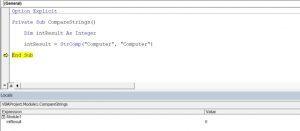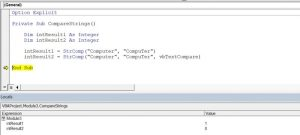# VBA Compare Strings – StrComp

In VBA, you can compare two strings using the StrComp function. In this tutorial, you will learn how to use this function with different comparison methods.

If you want to learn how to use VBA like operator to compare inexact matches, click here: VBA Like Operator.

If you want to learn how to use comparison operators, click here: VBA Comparison Operators – Not Equal to & More

## Using the StrComp Function to Compare Two Strings

The StrComp function takes two strings and comparison method as arguments. There are 3 methods of comparison:

• vbBinaryCompare – compares two strings binary (case-sensitive);
• vbTextCompare – compares two strings as texts (case-insensitive);
• vbDatabaseCompare – this method is available only in Microsoft Access and not in VBA.

By default, the function uses the binary method. If we omit this argument, the function will be case-sensitive.

The function returns the following results:

• 0 – if strings are equal;
• -1 – if string 1 is less than string 2;
• 1 – if string 1 is greater than string 2;
• Null – if one of the strings is empty.

Here is the example code:

``````Dim intResult As Integer

intResult = StrComp("Computer", "Computer")``````

In this example, we want to check if strings “Computer” and “Computer” are equal, using the default comparison method (binary). The result of the function is in the intResult variable. As you can see in Image, both strings are equal, therefore the intResult has value 0.Image 1. Using the StrComp function with the binary method in VBA

## Using the StrComp Function with Binary and Textual Method

You will now see the difference between using the binary and textual method. The binary method has a binary number for each character, so the uppercase and lowercase are different characters in this method. On the other side, the textual method considers “S” and “s” as the same letters. If you want to make the function case-insensitive, you need to set the third argument to vbTextCompare. Here is the code:

``````Dim intResult1 As Integer
Dim intResult2 As Integer

intResult1 = StrComp("Computer", "CompuTer")

intResult2 = StrComp("Computer", "CompuTer", vbTextCompare)``````

We want to compare strings “Computer” and “CompuTer”, using both methods.

In the variable intResult1, we will get the value with the binary method, while the intResult2 will get the value with the textual method. You can see the values of these two variables:Image 2. Using the StrComp function with binary and textual method

The value of intResult1 is 1, which means that two strings are different for the binary method. For the textual method, these two strings are equal, so the value of intResult2 is 0.

Stop searching for VBA code online. Learn more about AutoMacro - A VBA Code Builder that allows beginners to code procedures from scratch with minimal coding knowledge and with many time-saving features for all users!